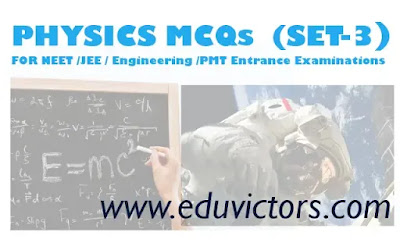## Pages

Showing posts with label PhysicsMCQs. Show all posts
Showing posts with label PhysicsMCQs. Show all posts

# CBSE Class 10 - Physics - Light - Reflection and Refraction (MCQs)

Q1: The laws of reflection hold true for:

(a) plane mirrors only

(b) concave mirrors only

(c) convex mirrors only

(d) all reflecting surfaces

Q2: Focal length of a plane mirror is

(a) zero

(b) infinite

(c) 25 cm

(d) -25 cm# PHYSICS MCQs FOR NEET - IIT JEE EXAMINATION (SET-6)

Topics Covered: Work, Power and Energy

Q1: A bullet is fired from a rifle, If the rifle recoils freely, then the kinetic energy of the rifle will be:

(a) same as that of bullet

(b) more than that of bullet

(c) less than that of bullet

(d) none of these

Q2: A body of mass 2 kg, initially at rest, is acted upon simultaneously by two forces, one of 4N and the other of 3N, acting at right angles to each other. The work done by the body after the 20s is

(a) 500 J

(b) 1250 J

(c) 2500 J

(d) 5000 J# JEE NEET PHYSICS MCQs (Set-5)

Topics Covered: Laws of Motion, Circular Motion

Q1: In a tug-of-war match, one team is pulling with a force of 500 N. If they are exactly balanced by the other team, then the tension in the rope is?

(a) 0
(b) 250 N
(c) 500 N
(d) 1000 N

Q2: A particle of mass 0.3 kg is subjected to a force F = kx with k= 15 N/m. What will be its initial acceleration if it is released from a point 20 cm away from the origin.

(a) 3 m/s²
(b) 15 m/s²
(c) 5m/s²
(d) 10 m/s²# JEE NEET PHYSICS MCQs (Set-4)

Topics Covered: Vector Algebra, Motion in a Plane

Q1: If θ is the angle between two vectors, then the resultant vector is maximum when the value of θ is

(a) 0°
(b) 90°
(c) 180°
(d) Same in all cases.

Q2: The change in a vector may occur due to

(a) Rotation of the frame of reference
(b) Translation of frame of reference
(c) Rotation of vector
(d) Both a and b

Q3: Acceleration of a particle under projectile motion at the highest point of its trajectory is :

(a) g
(b) zero
(c) less than g
(d) dependent upon projection velocity## PHYSICS MCQs (Set-3)

FOR JEE NEET Med/Engineering Entrance Examinations

Topics Covered: Kinematics (Motion in a Straight Line)

Q1: A particle starts from rest with uniform acceleration 'a' Its velocity after 'n' second is 'v'. The displacement in last two seconds is:

(a) 2v(n-1)/n
(b) v(n-1)/n
(c) v(n+1)/n
(d) 2v(2n+1)/n

Q2: A body starts from origin and moves along x-axis such that at any instant velocity is v = 4t³ - 2t. The acceleration of the particle when is it is 2m away from the origin is:

(a) 14 m/s²
(b) 18 m/s²
(c) 22 m/s²
(d) 32 m/s²## JEE NEET PHYSICS MCQs (Set-2)

FOR NEET - IIT JEE and Other Engineering or premedical Entrance Examinations

Topics Covered: Units & Dimensions, Error in Measurement

Q1: Which of the following sets cannot enter into the list of fundamental quantities in any system of unit

(a) length, mass and velocity
(b) length, time and velocity
(c) mass, time and velocity
(d) length, time and mass

Q2: The base quantity among the following is:

(a) Speed
(b) Weight
(c) Length
(d) Area## PHYSICS MCQs  (SET-1)

FOR NEET - IIT JEE and Other Engineering or premedical Entrance Examinations

Q1: A neutron with velocity v strikes a stationary deuterium atom, its kinetic energy changes by a factor of

(a) 15/16
(b) 1/2
(c) 2/1
(d) 8/9

Q2: AM radio band has carrier frequency from 500 kHz to 1500 kHz. Assuming a fixed inductance in a simple LC circuit, what is  ratio of highest capacitance to lowest capacitance required to cover this frequency range?

(a) 3
(b) 6
(c) 9
(d) 12